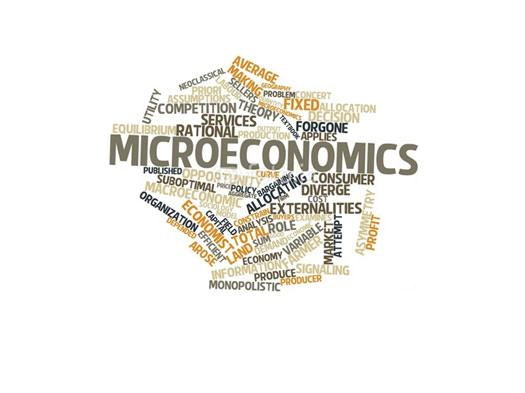# Microeconomics Trivia

26 QuestionsSettingsThe Costs of Production

Related Topics
• 1.
• A.

True

• B.

False

• 2.
Wages and salaries paid to workers are an example of implicit costs of production
• A.

True

• B.

False

• 3.
If total revenue is \$100, explicit costs are \$50, and implicit costs are \$30, then accounting profit equals \$50
• A.

True

• B.

False

• 4.
If there are implicit costs of production, accounting profits will exceed economic profits
• A.

True

• B.

False

• 5.
When a production function gets flatter, the marginal product is increasing
• A.

True

• B.

False

• 6.
If a firm continues to employ more workers within the same size factory, it will eventually experience diminishing marginal product
• A.

True

• B.

False

• 7.
If the production function for a firm exhibits diminishing marginal product, the corresponding total-cost curve for the firm will become flatter as the quantity of output expands
• A.

True

• B.

False

• 8.
Fixed cost plus variable costs equal total costs
• A.

True

• B.

False

• 9.
Average total costs are total costs divided by marginal costs
• A.

True

• B.

False

• 10.
When marginal costs are below average total costs, average total costs must be falling
• A.

True

• B.

False

• 11.
If, as the quantity produced increases, a production function first exhibits increasing marginal product and later diminishing marginal product, the corresponding marginal-cost curve will be U-shaped
• A.

True

• B.

False

• 12.
The average-total-cost curve crosses the marginal-cost curve at the minimum of the marginal-cost curve
• A.

True

• B.

False

• 13.
The average-total-cost curve in the long run is flatter than the average-total-cost curve in the short run
• A.

True

• B.

False

• 14.
The efficient scale for a firm is the quantity of output the minimizes marginal cost
• A.

True

• B.

False

• 15.
In the long run, as a firm expands its production facilities, it generally first experiences diseconomies of scale then constant returns to scale, and finally economies of scale
• A.

True

• B.

False

• 16.
Accounting profit is equal to total revenue minus
• A.

Implicit costs

• B.

Explicit costs

• C.

The sum of implicit and explicit costs

• D.

Marginal costs

• E.

Variable costs

• 17.
Economic profit is equal to total revenue minus
• A.

Implicit costs

• B.

Explicit costs

• C.

The sum of the implicit and explicit costs

• D.

Marginal costs

• E.

Variable costs

• 18.
If there are implicit costs of production
• A.

Economic profit will exceed accounting profit

• B.

Accounting profit will exceed economic profit

• C.

Economic profit and accounting profit will be equal

• D.

Economic profit will always be zero

• E.

Accounting profit will always be zero

• 19.
If a production function exhibits diminishing marginal product, its slope
• A.

Becomes flatter as the quantity of the input increases

• B.

Becomes steeper as the quantity of the input increases

• C.

Is linear (a straight line)

• D.

Could be any of the above

• 20.
• A.

Becomes flatter as the quantity of output increases

• B.

Become steeper as the quantity of output increases

• C.

Is linear (a straight line)

• D.

Could be any of the above

• 21.
Which of the following is a variable cost in the short run?
• A.

Wages paid to factory labor

• B.

Payment on the lease for factory equipment

• C.

Rent on the factory

• D.

Interest payments on borrowed financial capital

• E.

Salaries paid to upper management

• 22.
When marginal costs are below average total costs
• A.

Average fixed costs are rising

• B.

Average total costs are falling

• C.

Average total costs are are rising

• D.

average total costs are minimized

• 23.
If, as the quantity produced increases, a production function first exhibits increasing marginal product and later diminishing marginal product, the corresponding marginal-cost curve will
• A.

Slope upward

• B.

Be U-shaped

• C.

Slope downward

• D.

Be flat (horizontal)

• 24.
In the long run, if a very small factory were to expand its scale of operations, it is likely that it would initially experience
• A.

Economies of scale

• B.

Constant returns to scale

• C.

Diseconomies of scale

• D.

An increase in average total costs

• 25.
The efficient scale of production is the quantity of output that minimizes
• A.

Average total cost

• B.

Marginal cost

• C.

Average fixed cost

• D.

Average variable cost

• 26.
Which of the following statements is true
• A.

All costs are fixed in the long run

• B.

All costs are variable in the long run

• C.

All costs are fixed in the short run

• D.

All costs are variable in the short run